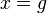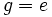# Nonempty finite subsemigroup of group is subgroup

## Contents

This article gives the statement, and possibly proof, of a basic fact in group theory.
View a complete list of basic facts in group theory
VIEW FACTS USING THIS: directly | directly or indirectly, upto two steps | directly or indirectly, upto three steps|

## Statement

### Verbal statement

Any nonempty multiplicatively closed finite subset (or equivalently, nonempty finite subsemigroup) of a group is a subgroup.

### Symbolic statement

Let$G$ be a group and$H$ be a nonempty finite subset such that$a,b \in H \implies ab \in H$. Then,$H$ is a subgroup of$G$.

## Proof

### Lemma

Statement of lemma: For any$x \in H$:

1. All the positive powers of$x$ are in$H$
2. There exists a positive integer$n(x)$, dependent on$x$, such that$x^{n(x)} = e$.

Proof:$H$ is closed under multiplication, so we get that the positive power of$x$ are all in$H$. This proves (1).

Since$H$ is finite, the sequence$x,x^2,x^3, \ldots$ must have some repeated element. Thus, there are positive integers$k > l$ such that$x^k = x^l$. Multiplying both sides by$x^{-l}$, we get$x^{k-l} = e$. Set$n(x) = k - l$, and we get$x^{n(x)} = e$. Since$k > l$,$n(x)$ is a positive integer.

### The proof

We prove that$H$ satisfies the three conditions for being a subgroup, i.e., it is closed under all the group operations:

• Binary operation: Closure under the binary operation is already given to us.
• Identity element$e \in H$: Since$H$ is nonempty, there exists some element$u \in H$. Set$x = u$ in the lemma. Applying part (2) of the lemma, we get that$e$ is a positive power of$u$, so by part (1) of the lemma,$e \in H$.
• Inverses$g \in H \implies g^{-1} \in H$: Set$x = g$ in the lemma. We make two cases:
• Case$n(g) = 1$: In this case$g = e$ forcing$g^{-1} = e = g \in H$.
• Case$n(g) > 1$: In this case$g^{-1} = g^{n(g) - 1}$ is a positive power of$g$, hence by Part (1) of the lemma,$g^{-1} \in H$.